Shi, G., Zhang, Y., Zhao, Y., Chao, L., and Cao, J. (2019). "Prediction methodology for wood compression strength based on particle-filtered near-infrared spectroscopy," BioRes. 14(4), 9704-9718.

#### Abstract

The measurement of wood mechanical properties is important for engineering design and applications. This study investigated near-infrared (NIR) spectroscopy coupled with particle filter (PF) and partial least-squares (PLS) methods to predict wood compression strength. Three structural timbers (Acer mono, birch, and toothed oak) were studied. The NIR spectra were collected from 900 to 1700 cm-1 and preprocessed by a standard normal variate transformation combined with Savitzky-Golay filtering. The prediction model coefficient matrix and standard variance were obtained by a PF iterative process, and their ratio was used to select the NIR feature wavelength points. A PLS prediction model based on NIR spectroscopy was established to predict the wood compression strength. Compared with the successive projection algorithm (SPA) and Kalman filtering (KF), the PF-PLS prediction model outperformed the other models in all three wood samples, resulting in a high correlation coefficient (r) of 0.89, 0.92, and 0.90, a low root-mean-square error of prediction (RMSEP) of 6.30, 10.60, and 9.71, and a fast average detection speed of 0.28 s, 0.46 s, and 0.33 s, respectively. The optimal PF selection can effectively reduce the redundant information of the NIR matrix and improve the accuracy and efficiency of the prediction model.

Prediction Methodology for Wood Compression Strength Based on Particle-Filtered Near-Infrared Spectroscopy

Guangyu Shi, Yizhuo Zhang, Yinong Zhao, Li Chao,* and Jun Cao *

The measurement of wood mechanical properties is important for engineering design and applications. This study investigated near-infrared (NIR) spectroscopy coupled with particle filter (PF) and partial least-squares (PLS) methods to predict wood compression strength. Three structural timbers (Acer mono, birch, and toothed oak) were studied. The NIR spectra were collected from 900 to 1700 cm-1 and preprocessed by a standard normal variate transformation combined with Savitzky-Golay filtering. The prediction model coefficient matrix and standard variance were obtained by a PF iterative process, and their ratio was used to select the NIR feature wavelength points. A PLS prediction model based on NIR spectroscopy was established to predict the wood compression strength. Compared with the successive projection algorithm (SPA) and Kalman filtering (KF), the PF-PLS prediction model outperformed the other models in all three wood samples, resulting in a high correlation coefficient (r) of 0.89, 0.92, and 0.90, a low root-mean-square error of prediction (RMSEP) of 6.30, 10.60, and 9.71, and a fast average detection speed of 0.28 s, 0.46 s, and 0.33 s, respectively. The optimal PF selection can effectively reduce the redundant information of the NIR matrix and improve the accuracy and efficiency of the prediction model.

Keywords: Wood compression strength; Near-infrared spectroscopy; Nonlinear dynamic system; Particle filter; Partial least-square

Contact information: Northeast Forestry University, Harbin, 150040, China;

* Corresponding authors: lichaonefuzyz@nefu.edu.cn; zdhcjnefu@126.com

INTRODUCTION

Compression strength is an important mechanical indicator for structural timber. Qualified compression strength ensures that wood materials have a high degree of structural performance and reliability. The traditional method used to measure timber compression strength requires small, standard, flawless samples. Measurements are carried out on a universal testing machine according to the national standard. This approach is harsh, time-consuming, laborious, and destructive (Tsuchikawa 2007). Moreover, it cannot meet the current requirements of wood processing (Rakotovololonalimanana et al. 2015).

Near-infrared (NIR) spectroscopy is a fast, easy, and nondestructive analysis method that is being used to evaluate various properties of organic materials. The wavelength range of the NIR spectral area is 770 to 2500 nm. The spectral response provides vibration information associated with functions groups comprising carbon, oxygen, and hydrogen, and is also related to their structural information. The NIR spectral absorption peaks of various molecular hydrogen groups are clearly different. These spectra allow the analyses of chemical, physical, and biological information associated with complex materials. In recent years, researchers have extensively used NIR spectroscopy to detect the mechanical properties of wood. For instance, Watanabe et al. (2012) used NIR spectroscopy to detect the longitudinal growth strain of three Sugi green logs, based on which they constructed a partial-least-squares (PLS) regression model. The average coefficient of determination was 0.61, and NIR spectroscopy was capable of evaluating the magnitude of longitudinal growth stresses on green logs. Schimleck et al. (2018) established a PLS regression model to predict several types of pine moduli of elasticity and moduli of rupture coupled with the sample raw NIR spectra. This model yielded a good performance and the coefficient of determination of 0.64 to 0.81. Watanabe et al. (2014) established an ANN prediction model to predict the timber surface drying stress with NIR spectroscopy, which yielded a coefficient of determination of 0.79.

However, NIR spectra have the characteristics of “high-dimensional, overlap, nonlinearity, and redundancy.” In the processing and analysis of the NIR spectra, the effective spectral information is weak, and it can be easily drowned out by a large amount of raw spectral noise. Accordingly, the accuracy of modeling is reduced when redundant information between the spectral bands is accounted for (Watanabe et al. 2014). Various feature selection methods have been used to reduce the dimensions of the NIR spectra. Commonly used feature selection methods include the correlation coefficient method, genetic algorithm (GA), simulated annealing algorithm (SAA), uninformative variable elimination (UVE), and the successive projection algorithm (SPA). The correlation coefficient method is mainly based on subjective threshold selection (Bin et al. 2017). For a small sample size and high-dimensional datasets, GA and SAA are subject to poor reliability and long operation times (Tripathi and Mishra 2009; Mahesh et al. 2015; Xu et al. 2016; Liang et al. 2016; Zareef et al. 2018). The uniformative variable elimination (UVE) is a wavelength selection algorithm based on partial least-squares regression coefficients, and it is used to eliminate variables that do not provide information (Liu et al. 2017). The successive projection analysis method (SPA) is a new variable extraction method. It can use vectorial projection analyses to identify a set of variables with minimum redundant information and minimize the collinearity between the variables (Liang et al. 2018).

Particle filter is a process of approximating a probability density function by finding a group of random samples propagating in the state space coupled with the Successive Monte Carlo simulation method, and replacing integral operation with a sample mean to obtain the minimum variance distribution of the state of a nonlinear dynamic system (Wang and Lu 2014). The random samples are referred to as “particles” vividly, so the algorithm is usually named as particle filtering. Because of the superiority of the particle filter technology in non-linear and non-Gaussian systems, its application range is very wide, such as visual tracking, object location, and nuclear medical imaging (Wang et al. 2011; Rahni et al. 2011; Yin and Zhu 2015). In this paper, wood NIR spectra features of compression strength and spectral processing method was studied. The PF-PLS prediction model was established for predicting the compression strength of wood, and three commonly used structural timbers (Acer mono, birch, and toothed oak) were evaluated. During the successive PF iterations, the contribution rate of each NIR wavelength point was calculated. Along with the rank of contribution rate of each NIR wavelength point, optimal NIR spectral feature wavelength points were selected. The prediction model was optimized and had a high predictive accuracy.

EXPERIMENTAL

Material Preparation and Compression Strength Measurement

Three commonly used structural timber types, i.e.Acer mono, birch, and toothed oak, were selected as experimental materials. Six logs of Acer mono, birch, and toothed oak were collected from the Dailing Forestry Bureau in the Heilongjiang Province, China. The logs were 1.3 m in height, and several discs had a thickness of 50 cm. According to the GB/T1928 (2009) standard, the discs were cut into qualified compression strength samples with the dimensions of 30 mm (L) × 20 mm (T) × 20 mm (R). After processing, 107 qualified Acer mono samples, 80 qualified birch samples, and 102 qualified toothed oak samples were obtained. The samples were placed in a thermostat box, and the temperature and relative humidity were recorded at 22 °C and at a relative humidity of 65% in an air-dry station. The rate of water content of the samples was maintained at 12%.

In accordance with standard GB/T 1935 (2009), the compression strength of the samples was tested on a universal strength testing machine. Each sample type was randomly divided into a calibration set and a test set according to the ratio of 2:1. The calibration set was used to select the feature variables and train the prediction model. The test set was used to assess the testing model performance.

NIR Spectral Measurements and Preprocessing

An NIR Quest (512) spectrometer was used to measure the sample surface in the wavelength range of 900 to 1700 nm at 3-nm intervals. The spectral content has been used to analyze the properties of wood in the wavelength range of 1100 to 1700 nm (Schimleck et al. 2003a; Todorović et al. 2015). A stable, 512-pixel, indium gallium arsenide array detector was located on a compact light pedestal with two-stage thermoelectric coolers and low-noise electronic components, and it was used to scan the surface of samples so that the errors attributed to improper operations could be eliminated effectively. After a 10-min preheating period, a calibration scan was executed on the polytetrafluoroethylene reference tile based on the LY/T 2053 (2012) standard. As the detector scanned the surface of the samples, the sample’s NIR spectral data were obtained with the software SPEC view 7.1 (Parr Instrument Company, Moline, IL, USA). The data were exported in Microsoft Excel software. Each sample was scanned 30 times, but one averaged NIR spectrum dataset was obtained.

For reducing the negative effects in the raw NIR spectrum collecting process, this study used a standard normal variate transformation (SNV) and a Savitzky-Golay (SG) smoothing filter to preprocess the raw NIR spectrum. SNV could improve the light scattering, and the Savitzky-Golay (SG) smoothing filter could improve the high-frequency noise and the spectral baseline drift problem. The SNV process was executed as follows,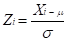(1)

where Xi is the original spectrum, μ is the mean value of the original spectrum, and σ is the standard deviation of the original spectrum.

The SG smoothing filter utilized a polynomial approach to construct a least-squares fitting methodology for use in moving windows. This indicated that the total number of wavelength points per spectrum was D, the wavelength point sequence number was j (j=1, 2, …, D), the width of the moving windows was 2m+1 (-m, -m+1, …m-1, m), and aj={a0, a1, … ak} was the weight coefficient, which describe a k-order polynomial,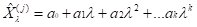(2)

where λ is the point absorbance of the moving window’s NIR wavelength.

In the moving window, the minimized error between the fitted (based on the use of polynomials) and the original NIR spectra was as follows,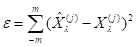(3)

where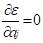. The corresponding weight coefficient combination was calculated to yield the smallest error for windows with different sizes.

Feature Wavelength Point Selection Based on PF

The PF estimates the current state of the dynamic system by combining the previously estimated system state with the observed value of the system (Yang et al. 2015). In this study, the sample NIR spectra and the corresponding compression strength were regarded as a nonlinear dynamic system. The PLS was selected as the prediction model. The PLS coefficients matrix was regarded as the system state, while the compression strength was regarded as the observed value. In the iterative PF process, the coefficient matrix was continually updated. The coefficient matrix of the PLS was denoted as bx as the NIR spectral data, and y as the corresponding compression strength value. The wood NIR spectroscopy compression strength dynamic system model function was denoted as follows,

State function: bk + 1 bk + wk (4)

Observation function: yk = xkbk + vk (5)

where is the iteration number, and random signal wk and random signal vk are the process incentive and observation noise, respectively.

Bn is the entire set of all the goal state sequences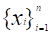. Similarly, Yn represents the observation sequence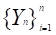. Accordingly, when Yn is known, all the goal state sequence joint posterior distribution can be denoted as shown in Eq. 6.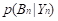(6)

Given that a dynamic system state changes follow to a Markov process (Yang et al. 2016), h(bn) denoted a random function of state Bn. Accordingly, the universal mean of h(bn) can be denoted as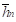, whilerepresents the signal character of the goal state sequences Bn. Based on the Bayesian estimation,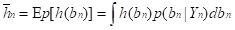(7)

where Ep is the desired value of the posterior distribution. Because Bn could not be observed, it was not feasible to sample randomly from posterior distribution directly. Accordingly, a new distribution was set and denoted as the instrumental distribution.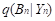(8)

where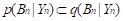. (9)

N statistically independent samples were randomly sampled from the instrumental distribution. The acquired sample set at time n is denoted as follows.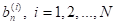(10)

These samples are acquired from the initial time to time n in a step-by-step manner and tracked a trajectory based on every observation at each iteration. These samples are also called particles. We can express the important function based on Eq. 8 as follows.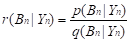(11)

Substituting Eq. 11 in Eq. 7 leads to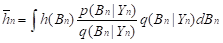(12)

Using the sampling method for the Bayesian estimator of Eq. 12, the corresponding Monte Carlo estimator is as follows,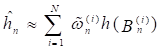(13)

where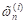denotes the importance weight and can be expressed as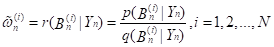(14)

According to the iterations of the observed values, the weights of the particles were changed, the particles with large weights were retained, and the particles with minimum weight were eliminated. To supplement new particles, the sampling importance resampling (SIR) method was used to resample particles from the retained particles (Arulampalam et al. 2002). PF reproduced particles from the remaining particles according to their weight ratio. At the end of the iteration, the optimal estimation of the system state at the current time was described according to the trajectories of the remaining particles.

This PF-PLS prediction model allowed the estimation of the model coefficient matrix b and the estimation error covariance matrix P. The ratio of the model coefficient matrix and standard deviation of the posterior estimation error was denoted as Rpf, where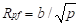(15)

A higher correlation degree of wavelength points resulted in a larger the corresponding weight of the model coefficient matrix b. As the standard deviation of the posterior estimation error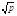decreases, the corresponding weights of the model coefficient matrix b become more accurate. According to the value of each NIR spectral feature wavelength point Rpf, ranked from high to low values, different numbers of wavelength points were selected and input to the prediction model.

When the PLS prediction model yielded the best performance, the desired wavelength points were selected, and the PF-PLS prediction was established. Figure 1 shows the workflow of the PF-PLS prediction model for the compression strength of wood.

Model Evaluation Standard

The model’s predictive performance was assessed using several common statistical measures (Schimleck et al. 2003b), including the root-mean-square error of prediction (RMSEP) and the correlation coefficient (r). In general, a good model has high r and low RMSEP values. Furthermore, the detected efficiency is also of interest. The average detection time for each sample was also recorded.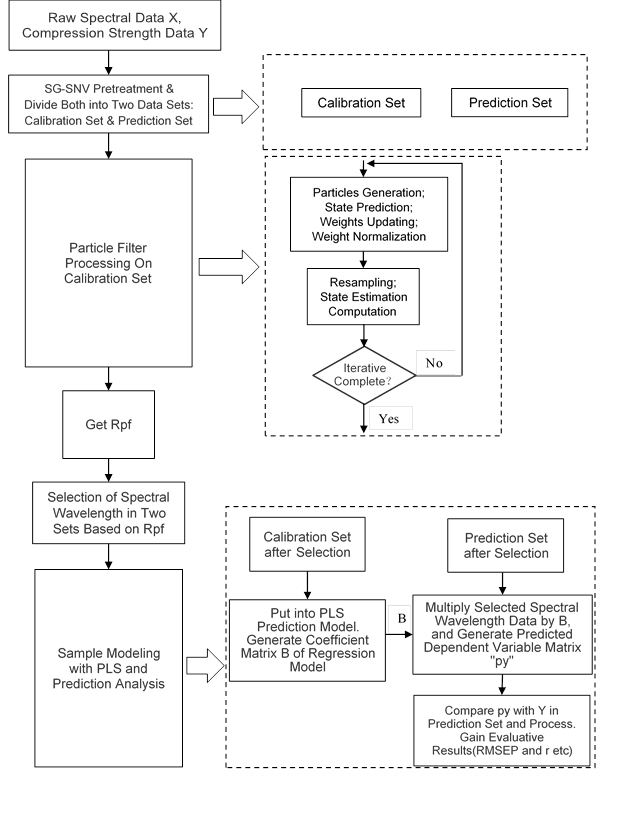Fig. 1. Flow chart of the PF-PLS prediction model

RESULTS AND DISCUSSION

In this study, Acer mono was used as an example to introduce the method in detail, and the simulation environment was based on the use of dedicated hardware (Intel(R) Core(TM) i5-8265U CPU @ 1.60 GHz 1.8 GHz) and specific software (Matlab R2018a).

Wood Compression Strength Determination

Samples were divided into the calibration and test sets in the ratio of 2:1. The sample compression strengths are listed in Table 1.

Table 1. Statistics of Compression Strength Based on the Calibration and Test Sets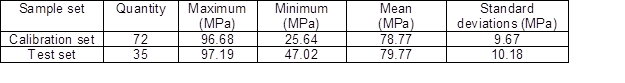Near-infrared Spectra of Samples and Spectral Preprocessing

The raw NIR spectra of samples were collected in the range of 899.77 to 1720.81 nm. Accordingly, 512 NIR spectral wavelength points were obtained. The raw NIR spectra of Acer mono samples are shown in Fig. 2.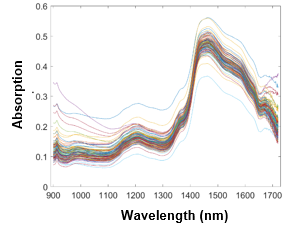Fig. 2. Raw NIR spectra of tested samples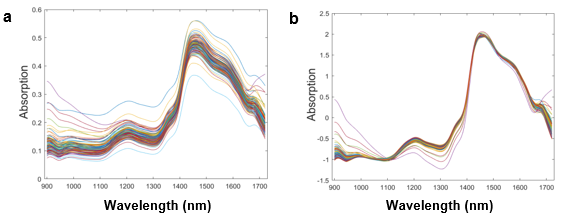Fig. 3. Preprocessed spectra: (a) Pretreated by SNV, (b) preprocessing by SNV combined with the SG convolution smoothing filter

The raw NIR spectral data were preprocessed by SNV and by an SG smoothing filter. When the order of the SG smoothing polynomial was equal to seven, and the width of the window was equal to nine, the absorption peak was concentrated, and the spectrum was smooth. The preprocessing results are shown in Fig. 3. Based on the comparison of Figs. 2 and 3, the light scattering weakened, and the change was more uniform after SNV processing. In contrast, the main absorption peaks of the spectrum were more evident, and the spectral profiles were smoother after SG filtering.

NIR Spectral Feature Points Selected Based on PF

After preprocessing, the 512 NIR spectral wavelength points of the calibration set were considered as optimized targets, and the compression strength values of the 72 Acer mono samples were considered as the iteration samples. The number of particles was set to be in the range of 5 to 80 according to the best performance of the PLS model. The variance of the system state noise was set to 10%, and the system measurement noise variance was set to 300%.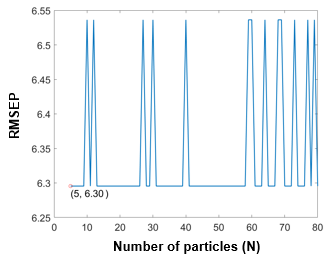Fig. 4. PF-PLS prediction model performance as a function of the number of particles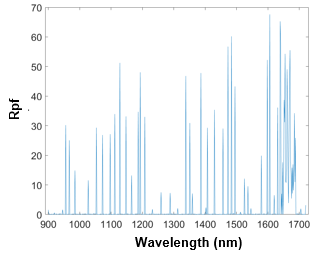Fig. 5. Feature selection result based on PF

After 72 iterations, the Rpf values of all the wavelength point were calculated and sorted in ascending order. Higher Rpf values represented a higher degree of importance of feature wavelength points in the model’s establishment. Figure 4 shows that when the number of particles is equal to five, PF yields the best performance. Figure 5 shows the Rpf values of all the feature wavelength points. Table 2 lists the top 12 most important feature wavelength points.

Table 2. Near-infrared (NIR) Feature Wavelength Points Ranked According to their Degree of Influence Based on Rpf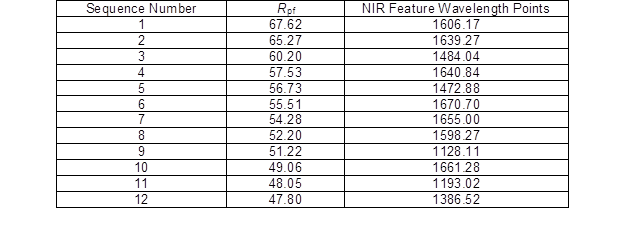Model Establishment and Performance Comparison

Different numbers of wavelength points were input to the PLS prediction model according to theirvalues. The root-mean-square error of the prediction was used to evaluate the prediction performance of PLS. Figure 6 shows the variation of RMSEP as a function of the number of variables. When the number of variables increased, the predicted RMSEP values of the PLS model gradually reduced. When the number of variables was equal to 11, the model’s RMSEP value attained its minimum value. Thus, the top 11 wavelength points in Table 2 were selected as the feature wavelengths. In addition, the PF-PLS compression strength prediction model for Acer mono yielded RMSEP = 6.30, a correlation coefficient r = 0.89, and an average detection speed for each sample of 0.28 s. In Fig. 7, the squares mark the variable positions of the selected wavelength points. Figure 8 presents the fitting degree between the predicted value and the real value.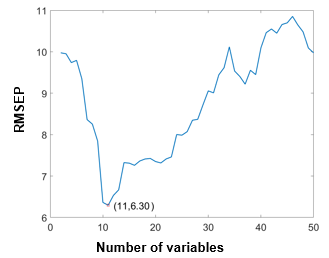Fig. 6. Predicted errors as a function of the number of variables based on PF-PLS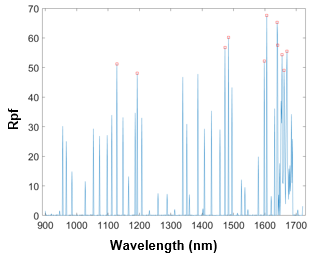Fig. 7. Variable positions of the selected wavelength points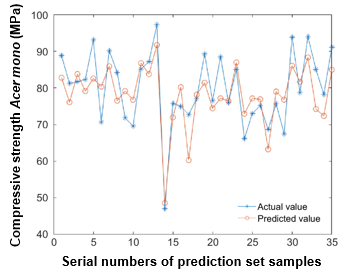Fig. 8. Actual and predicted values of the compressive strength of the Acer mono prediction set based on PF-PLS

The performances of the successive projection algorithm (SPA) and Kalman filtering (KF) for the extraction of the NIR spectral feature wavelength points were compared for the tested samples. SPA aims to identity the least information variable group from the spectral variables, and to reduce the collinearity between the variables of the variable group. Figure 9 shows the variation of RMSEP of the SPA-PLS model after the selection of the SPA variables for different numbers of feature wavelength points. When 13 variables were used, the RMSEP value of the SPA-PLS reached its minimum value. The SPA-PLS prediction model yielded RMSEP = 10.05 and r = 0.65, and an average detection speed for the samples of 8.21 s. Figure 10 presents the fitting degree between the predicted and the real values of the SPA-PLS prediction model.

KF is a recursive linear filter. In the estimation of the system state, KF assumes that both the system and the observation noise follow the Gauss distribution. Because the KF is easily calculated, it is often used in engineering practice.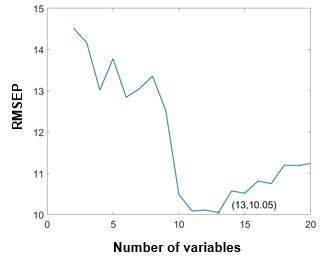Fig. 9. Variation of RMSEP of PLS as a function of the number of variables based on SPA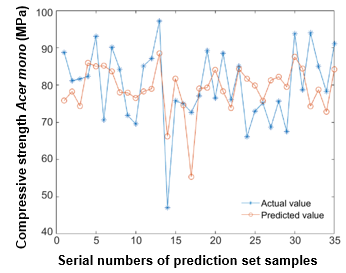Fig. 10. Actual and predicted values of the compressive strength of the Acer mono prediction set based on SPA-PLS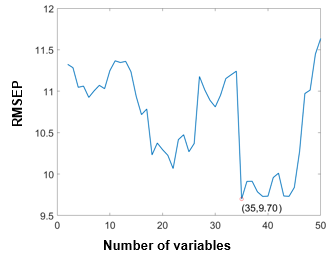Fig. 11. Variation of RMSEP of PLS as a function of the number of variables based on KF

Figure 11 shows the KF wavelength point selection results. Thirty-five feature wavelength points were selected by KF. Figure 12 shows the actual and predicted values of the KF-PLS prediction model. The KF-PLS model yielded RMSEP = 9.70, r = 0.76, and an average detection speed for the samples of 0.40 s.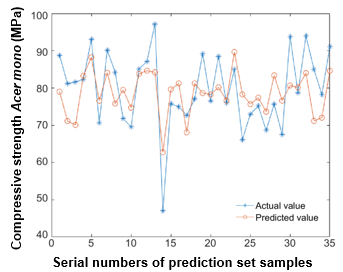Fig. 12. Actual value and predicted values of the compressive strength of the Acer mono prediction set based on KF-PLS

Table 3 lists the outcomes of the three aforementioned feature wavelength point methods and their effects on the PLS prediction models. Similarly, Tables 4 and 5 list the comparison outcomes for the compression strengths of the birch and toothed oak samples according to the predictive performance of the PLS model based on the three feature wavelength-point selection method.

Table 3. Comparison of Prediction Performances of Different PLS Models for the Compression Strength of Acer mono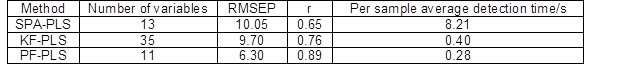Table 4. Comparison of Different Birch Compression Strengths Based on the Prediction Performance of the PLS Model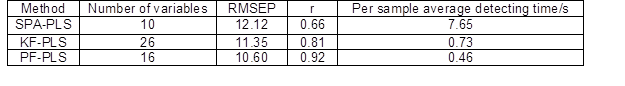Table 5. Comparisons of Different Toothed Oak Compression Strengths Based on the Predictive Performance of the PLS Model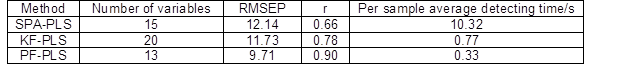Based on the comparison of the SPA with PF and KF, the solution of the dynamic system optimization problem of the NIR spectral wavelength point selection process is efficient. The comparison of KF with PF shows that the nonlinear optimization process of PF is more effective in the selection of useful feature wavelength points because the distribution of redundant NIR wavelength points is scattered, and it does not follow the Gaussian distribution. The selection of NIR spectral wavelength points based on PF, and the PLS prediction model for the compression strength of wood yielded fast and accurate performances.

CONCLUSIONS

1. Since the relationship between wood compression strength and its corresponding NIR spectra is not a simple linear function, a nonlinear dynamic system that used partial filter and PLS algorithms was established to fit this relationship. The experiment results shows that this nonlinear algorithm could optimize the performance of the prediction model.
2. The correlation coefficient, RMSEP and predictive time of three woods (Acer mono, birch, and toothed oak) by PF-PLS prediction model were 0.89, 0.92, 0.90; 6.30, 10.60, 9.71; and 0.28s, 0.46s, 0.33s, respectively, which shows that this PF NIR spectral feature wavelength points optimal selection methodology possesses a wide adaptation range in wood compression strength prediction.

AUTHOR CONTRIBUTIONS

For this research article, Guangyu Shi, Jun Cao and Chao Li conceived and designed the experiments; Yinong Zhao and Guangyu Shi performed the experiments; Guangyu Shi and Yizhuo Zhang analyzed the data; Guangyu Shi and Chao Li wrote the paper.

ACKNOWLEDGMENTS

The authors thank the Program of National Natural Science Foundation of China (CN) [Grant No. 31700643], National Forestry Bureau of 948 Project [Grant No. 2015-4-25), Postdoctoral Fund of Heilongjiang [Grant No. LBH-Q18006], and the Fundamental Research Funds for the Central Universities [Grant No. 2572015AB24].

CONFLICTS OF INTEREST

The authors declare no conflict of interest.

REFERENCES CITED

Arulampalam, M. S., Maskell, S., Gordon, N., and Clapp, T. (2002). “A tutorial on particle filters for online nonlinear/non-Gaussian Bayesian tracking,” IEEE Transactions on Signal Processing 50(2), 174-188. DOI: 10.1109/78.978374

Bin, J., Fan, W., Zhou, J. H., Li, X., and Liang, Y. Z. (2017). “Application of intelligent optimization algorithms to wavelength selection of near-infrared spectroscopy,” Guang Pu Xue Yu Guang Pu Fen Xi 37(1), 95-102.

GB/T 1928 (2009). “Wood physical and mechanical specimen collection method,” Standardization Administration of China, Beijing, China.

GB/T 1935 (2009). “Method of testing in compressive strength parallel to grain of wood” Standardization Administration of China, Beijing, China.

Liang, H., Cao, J., Tu, W., Lin, X., and Zhang, Y. (2016). “Nondestructive determination of the compressive strength of wood using near-infrared spectroscopy,” BioResources 11(3), 7205-7213. DOI: 10.15376/biores.11.3.7205-7213

Liang, H., Zhang, M., Gao, C., and Zhao, Y. (2018). “Non-destructive methodology to determine modulus of elasticity in static bending of Quercus mongolica using near-infrared spectroscopy,” Sensors 18(6), 1963. DOI: 10.3390/s18061963

Liu, X. W., Cui, X. Y., Yu, X. M., Cai, W. S., and Shao, X. G. (2017). “Understanding the thermal stability of human serum proteins with the related near-infrared spectral variables selected by Monte Carlo-uninformative variable elimination,” Chinese Chemical Letters 28(7), 1447-1452. DOI: 10.1016/j.cclet.2017.03.021

LY/T 2053 (2012). “Standard method for near infrared qualitative analysis of wood,” State Forestry Administration, Beijing, China.

Mahesh, S., Jayas, D. S., Paliwal, J., and White, N. D. G. (2015). “Comparison of partial least squares regression (PLSR) and principal components regression (PCR) methods for protein and hardness predictions using the near-infrared (NIR) hyperspectral images of bulk samples of Canadian wheat,” Food and Bioprocess Technology 8(1), 31-40. DOI:10.1007/s11947-014-1381-z

Rahni, A. A., Lewis, E., Guy, M. J., Goswami, B., and Wells, K. (2011). “A particle filter approach to respiratory motion estimation in nuclear medicine imaging,” IEEE Transactions on Nuclear Science 58(5), 2276-2285. DOI:10.1109/TNS.2011.2162158

Rakotovololonalimanana, H., Chaix, G., Brancheriau, L., Ramamonjisoa, L., Ramananantoandro, T., and Thevenon, M. F. (2015). “A novel method to correct for wood MOE ultrasonics and NIRS measurements on increment cores in Liquidambar styraciflua L.,” Annals of Forest Science 72(6), 753-761. DOI:10.1007/s13595-015-0469-6

Schimleck, L. R., Doran, J. C., and Rimbawanto, A. (2003a). “Near infrared spectroscopy for cost effective screening of foliar oil characteristics in a Melaleuca cajuputi breeding population,” Journal of Agricultural and Food Chemistry 51(9), 2433-2437. DOI:10.1021/jf020981u

Schimleck, L. R., Mora, C., and Daniels, R. F. (2003b). “Estimation of the physical wood properties of green Pinus taeda radial samples by near infrared spectroscopy,” Canadian Journal of Forest Research 33(12), 2297-2305. DOI:10.1139/x03-173

Schimleck, L. R., Matos, J. L. M., Trianoski, R., and Prata, J. G. (2018). “Comparison of methods for estimating mechanical properties of wood by NIR spectroscopy,” Journal of Spectroscopy. DOI:10.1155/2018/4823285

Todorovi, N., Popovi, Z., and Mili, G. (2015). “Estimation of quality of thermally modified beech wood with red heartwood by FT-NIR spectroscopy,” Wood Science and Technology 49(3), 527-549. DOI:10.1007/s00226-015-0710-3

Tripathi, S., and Mishra, H. N. (2009). “A rapid FT-NIR method for estimation of aflatoxin B1 in red chili powder,” Food Control 20(9), 840-846. DOI:10.1016/j.foodcont. 2008.11. 003

Tsuchikawa, S. (2007). “A review of recent near infrared research for wood and paper,” Applied Spectroscopy Reviews 42(1), 43-71. DOI: 10.1080/05704920601036707

Wang, F., Guo, Q., and Lin, Y. (2011). “A Kalman particle filter for bearing-only target tracking,” Journal of Computational Information Systems 7(15), 5628-5635.

Wang, F., and Lu, M. (2014). “Robust particle tracker via Markov Chain Monte Carlo posterior sampling,” Multimedia Tools and Applications 72(1), 573-589. DOI:10.1007/s11042-013-1379-y

Watanabe, K., Kobayashi, I., Matsushita, Y., Saito, S., Kuroda, N., and Noshiro, S. (2014). “Application of near-infrared spectroscopy for evaluation of drying stress on lumber surface: A comparison of artificial neural networks and partial least squares regression,” Drying Technology 32(5), 590-596. DOI:10.1080/07373937.2013.846911

Watanabe, K., Yamashita, K., and Noshiro, S. (2012). “Non-destructive evaluation of surface longitudinal growth strain on Sugi (Cryptomeria japonica) green logs using near-infrared spectroscopy,” Journal of Wood Science 58(3), 267-272. DOI:10.1007/s10086-011-1238-2

Yang, T., Mehta, P. G., and Meyn, S. P. (2015). “Feedback particle filter for a continuous-time Markov chain,” IEEE Transactions on Automatic Control 61(2), 556-561. DOI:10.1109/TAC.2015.2444171

Yin, S., and Zhu, X. (2015). “Intelligent particle filter and its application to fault detection of nonlinear system,” IEEE Transactions on Industrial Electronics 62(6), 3852-3861. DOI:10.1109/TIE.2015.2399396

Zareef, M., Chen, Q., Ouyang, Q., Kutsanedzie, F. Y., Hassan, M. M., Viswadevarayalu, A., & Wang, A. (2018). “Prediction of amino acids, caffeine, theaflavins and water extract in black tea using FT-NIR spectroscopy coupled chemometrics algorithms,” Analytical Methods 10(25), 3023-3031. DOI:10.1039/C8AY00731D

Article submitted: May 20, 2019; Peer review completed: August 25, 2019; Revised version received and accepted: October 18, 2019; Published: October 23, 2019.

DOI: 10.15376/biores.14.4.9704-9718